### Home > A2C > Chapter 10 > Lesson 10.1.2 > Problem10-45

10-45.
1. Change the angle measurements below from radians to degrees or from degrees to radians. Homework Help ✎

1. 144°

2. 300°

3.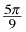4.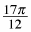5.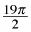6. 220°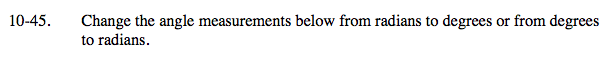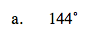$144\degree \cdot \frac{\pi}{180\degree}$

$\frac{4\pi}{5}$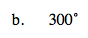See part (a).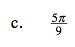$\frac{5\pi}{9}\cdot \frac{180\degree}{\pi}$

100°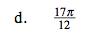See part (c).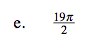See part (c).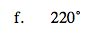See part (c).